# How to approach this?

#### allegansveritatem

##### Full Member
Here is the problem: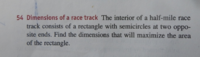I really couldn't come at this in any way. There seems to be not enough information. What I finally did was try to find the radius as a function of ....I'm not even sure of what but I called it X. This is what I tried: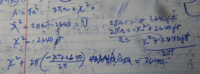This came to nothing. It was a hard way tautology. So then I tried a graph: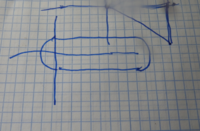This also didn't seem to give me any purchase on this problem. So...what to do?

#### Subhotosh Khan

##### Super Moderator
Staff member
Here is the problem:
View attachment 13196

I really couldn't come at this in any way. There seems to be not enough information. What I finally did was try to find the radius as a function of ....I'm not even sure of what but I called it X. This is what I tried:
View attachment 13197

This came to nothing. It was a hard way tautology. So then I tried a graph:
View attachment 13198

This also didn't seem to give me any purchase on this problem. So...what to do?
Let the length of the rectangular part =L

Width of the rectangular part = D

Then:

2 * L + pi * D = 2640 ft. ................ L = (2640 - pi*D)/2

Area included by the track A = L * D + pi/4 * D^2 = D * (2640 - pi*D)/2 + pi/4 * D^2 ................edited

continue.....

Last edited:

#### Dr.Peterson

##### Elite Member
Here is the problem:
View attachment 13196

I really couldn't come at this in any way. There seems to be not enough information. What I finally did was try to find the radius as a function of ....I'm not even sure of what but I called it X.
Don't miss the fact that they want you to maximize the area of the rectangle, not of the entire area inside the track.

Also, don't write any expressions until you know what your variable means, or you are sure to make mistakes. There is no square here with side X. There is a rectangle with two unknown dimensions (L and D if you like, or L and W, or whatever). Defining the variables is far more important than naming them.

#### pka

##### Elite Member
The instructions are to: Find the dimensions that will maximize the area of the rectangle.
The area of the rectangle is length times width: $$\displaystyle \large A=L\cdot W$$.
Now the length of the whole track is $$\displaystyle 2L+2W+\pi\cdot W=2640$$
Solving for $$\displaystyle L=\frac{2640-2W-\pi\cdot W}{2}$$.
Now maximize $$\displaystyle A$$.

#### Subhotosh Khan

##### Super Moderator
Staff member
Don't miss the fact that they want you to maximize the area of the rectangle, not of the entire area inside the track.

Also, don't write any expressions until you know what your variable means, or you are sure to make mistakes. There is no square here with side X. There is a rectangle with two unknown dimensions (L and D if you like, or L and W, or whatever). Defining the variables is far more important than naming them.
Oooops, I did miss that fact

#### allegansveritatem

##### Full Member
Don't miss the fact that they want you to maximize the area of the rectangle, not of the entire area inside the track.

Also, don't write any expressions until you know what your variable means, or you are sure to make mistakes. There is no square here with side X. There is a rectangle with two unknown dimensions (L and D if you like, or L and W, or whatever). Defining the variables is far more important than naming them.
I think what I was trying to do is see how this problem would work out if I had a square and a circle to work with...I guess I was trying to get a quadratic equation the quickest way I could. Pretty crazy, I know, but these word problems have a way of crazing a body.
Part of my problem, I think, is I am weak in geometry; otherwise I would have seen right away that side W is equal to 2 r. Geometry isn't just for little kids anymore!

Last edited:

#### allegansveritatem

##### Full Member
The instructions are to: Find the dimensions that will maximize the area of the rectangle.
The area of the rectangle is length times width: $$\displaystyle \large A=L\cdot W$$.
Now the length of the whole track is $$\displaystyle 2L+2W+\pi\cdot W=2640$$
Solving for $$\displaystyle L=\frac{2640-2W-\pi\cdot W}{2}$$.
Now maximize $$\displaystyle A$$.
I will try this. Thanks. You are saying that we want L(w), no?

#### pka

##### Elite Member
So you get L as a function of w? Or L(w)= etc. ? I will try this.
I will encourage you to see $$\displaystyle A(W)=\frac{2640W-2W^2-\pi\cdot W^2}{2}$$
Make $$\displaystyle A(W)$$ maximum.

#### allegansveritatem

##### Full Member
I will encourage you to see $$\displaystyle A(W)=\frac{2640W-2W^2-\pi\cdot W^2}{2}$$
Make $$\displaystyle A(W)$$ maximum.
I did use your tip ...but here is what I did with it: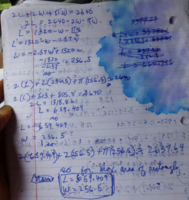I found L(w), Found the x(w) component of the resulting quadratic and plugged it into the equation for the perimeter and got L. Yes? No? Wait...now that I think about it the function I got was not l(w), it was A(W) so I guess I did exactly as suggested.

Last edited:

#### Dr.Peterson

##### Elite Member
Unfortunately, you used an incorrect formula from here:
The area of the rectangle is length times width: $$\displaystyle \large A=L\cdot W$$.
Now the length of the whole track is $$\displaystyle 2L+2W+\pi\cdot W=2640$$
Solving for $$\displaystyle L=\frac{2640-2W-\pi\cdot W}{2}$$.
Now maximize $$\displaystyle A$$.
The length of the track does not include the ends of the rectangle, but is just $$\displaystyle 2L+\pi\cdot W=2640$$.

This will change your results, and make some of the work easier.

Looking at your work, you have written a lot more than is necessary; and you used decimals all the way through. I would leave pi as a symbol until the final evaluations, which will save work and risk, as well as avoiding rounding. You also wrote L where you meant A at one point, and you seem to have used -b/a where I would expect you to use -b/(2a).

But you are close to the end.

#### allegansveritatem

##### Full Member
Unfortunately, you used an incorrect formula from here:

The length of the track does not include the ends of the rectangle, but is just $$\displaystyle 2L+\pi\cdot W=2640$$.

This will change your results, and make some of the work easier.

Looking at your work, you have written a lot more than is necessary; and you used decimals all the way through. I would leave pi as a symbol until the final evaluations, which will save work and risk, as well as avoiding rounding. You also wrote L where you meant A at one point, and you seem to have used -b/a where I would expect you to use -b/(2a).

But you are close to the end.
Well, the track is described as "half-mile". So I don't see how I could get L out of an equation that included the figure 2640 without including the pi diameter element. Am I wrong?
I translated pi into a decimal as a way of getting rid of one of the w^2's. Seemed like a good idea at the time.
It does look like I did not multiply the 2.57 by two but it seems to me I did and got something like 5.14. And 1320/5.14 is 256.8 which is what I got (actually I have 256.5). The above work I posted is actually a clean copy of the stuff I did today on this problem. I spent all my math time today on this guy (2hrs) and I think I tried about everything at least once. But I will go over it again tomorrow and make a more intelligible post of the results.

#### pka

##### Elite Member
Well, the track is described as "half-mile". So I don't see how I could get L out of an equation that included the figure 2640 without including the pi diameter element. Am I wrong? I translated pi into a decimal as a way of getting rid of one of the w^2's. Seemed like a good idea at the time.
It does look like I did not multiply the 2.57 by two but it seems to me I did and got something like 5.14. And 1320/5.14 is 256.8 which is what I got (actually I have 256.5). The above work I posted is actually a clean copy of the stuff I did today on this problem. I spent all my math time today on this guy (2hrs) and I think I tried about everything at least once. But I will go over it again tomorrow and make a more intelligible post of the results.
I am ashamed of myself missing the length of the track. Its length is $$\displaystyle 2L+W\cdot\pi=2640$$.
The area to be maximize is $$\displaystyle A=L\cdot W$$ so solve for $$\displaystyle L=\frac{2640-W\cdot\pi}{2}$$
Thus $$\displaystyle A(W)=L\cdot W=\frac{2640\cdot W-W^2\cdot\pi}{2}$$
Can you maximize $$\displaystyle A(W)~?$$

Last edited:

#### Dr.Peterson

##### Elite Member
Well, the track is described as "half-mile". So I don't see how I could get L out of an equation that included the figure 2640 without including the pi diameter element. Am I wrong?
I translated pi into a decimal as a way of getting rid of one of the w^2's. Seemed like a good idea at the time.
It does look like I did not multiply the 2.57 by two but it seems to me I did and got something like 5.14. And 1320/5.14 is 256.8 which is what I got (actually I have 256.5). The above work I posted is actually a clean copy of the stuff I did today on this problem.
I didn't say there's no pi in the problem! I said to keep it written as pi, not 3.14159 or whatever, to save work. Look at pka's (corrected) formula; he has a pi in there, not a decimal. Just keep it looking like that until the end. But this is not required; it's just standard practice in working with problems like this, to save work. You'll get the right answer regardless. And you'll find the details easier when you use the right formula to begin with.

Just keep at it, and maybe when you have it finished, one of us can show you how we would write out the work, just to expose you to helpful techniques.

#### allegansveritatem

##### Full Member
I am ashamed of myself missing the length of the track. Its length is $$\displaystyle 2L+W\cdot\pi=2640$$.
The area to be maximize is $$\displaystyle A=L\cdot W$$ so solve for $$\displaystyle L=\frac{2640-W\cdot\pi}{2}$$
Thus $$\displaystyle A(W)=L\cdot W=\frac{2640\cdot W-W^2\cdot\pi}{2}$$
Can you maximize $$\displaystyle A(W)~?$$

Good. I missed that entirely. The extra 2W has nothing to do with the perimeter! So now I will go back to the drawing board for the third time.

#### allegansveritatem

##### Full Member
I didn't say there's no pi in the problem! I said to keep it written as pi, not 3.14159 or whatever, to save work. Look at pka's (corrected) formula; he has a pi in there, not a decimal. Just keep it looking like that until the end. But this is not required; it's just standard practice in working with problems like this, to save work. You'll get the right answer regardless. And you'll find the details easier when you use the right formula to begin with.

Just keep at it, and maybe when you have it finished, one of us can show you how we would write out the work, just to expose you to helpful techniques.
Well, I again went over this today but did not do right in that I still got the perimeter formula wrong. I know you pointed this out yesterday but it didn't register where it should have registered. So, I will redo the thing tomorrow and I will try to leave pi untranslated if I can.

#### allegansveritatem

##### Full Member
I didn't say there's no pi in the problem! I said to keep it written as pi, not 3.14159 or whatever, to save work. Look at pka's (corrected) formula; he has a pi in there, not a decimal. Just keep it looking like that until the end. But this is not required; it's just standard practice in working with problems like this, to save work. You'll get the right answer regardless. And you'll find the details easier when you use the right formula to begin with.

Just keep at it, and maybe when you have it finished, one of us can show you how we would write out the work, just to expose you to helpful techniques.
I think I have it now (or have I?):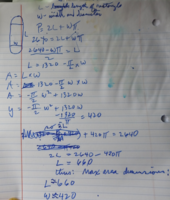#### Dr.Peterson

##### Elite Member
Looks good.

Interestingly, although 420 is approximate (rounded from 420.169...), if you leave W in exact form while solving for L, you get $$\displaystyle L = 1320 - \frac{\pi}{2}\frac{1320}{\pi} = 1320 - 660 = 660$$ exactly!

•JeffM

#### JeffM

##### Elite Member
There are FOUR reasons to keep irrational numbers in exact form until the very end.

The most important is to avoid build up of error. When you give a decimal approximation of any irrational number, you introduce an error into every subsequent calcultion. Those errors may accumulate and render your final approximation quite different from the true answer. So you will get the best approximation by doing all approximations as your final step.

Also, it is easier, as Dr. P said, and less error prone to work with the exact $$\displaystyle \pi ^3$$ than with the approximation of $$\displaystyle 31.0063.$$

And finally, in some cases, of which this is an example as Dr. P has shown, you may miss an opportunity to eliminate the irrational number altogether at a later stage of the calculation and then to give an exact answer rather than an approximate one.

Last edited:

#### allegansveritatem

##### Full Member
There are FOUR reasons to keep irrational numbers in exact form until the very end.

The most important is to avoid build up of error. When you give a decimal approximation of any irrational number, you introduce an error into every subsequent calcultion. Those errors may accumulate and render your final approximation quite different from the true answer. So you will get the best approximation by doing all approximations as your final step.

Also, it is easier, as Dr. P said, and less error prone to work with the exact $$\displaystyle \pi ^3$$ than with the approximation of $$\displaystyle 31.0063.$$

And finally, in some cases, of which this is an example as Dr. P has shown, you may miss an opportunity to eliminate the irrational number altogether at a later stage of the calculation and then to give an exact answer rather than an approximate one.
I get your point and take it.

#### allegansveritatem

##### Full Member
Looks good.

Interestingly, although 420 is approximate (rounded from 420.169...), if you leave W in exact form while solving for L, you get $$\displaystyle L = 1320 - \frac{\pi}{2}\frac{1320}{\pi} = 1320 - 660 = 660$$ exactly!
It is a simple problem, but not so simple if you don't think to connect w with 2r.